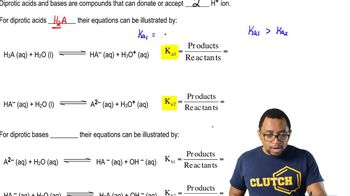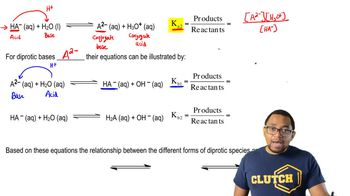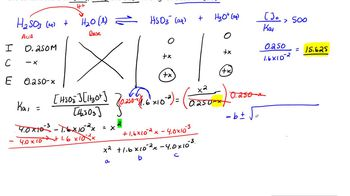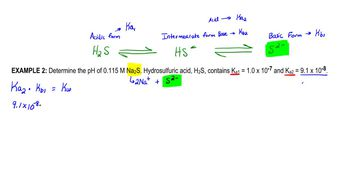Start typing, then use the up and down arrows to select an option from the list.# Diprotic Acids

Jules Bruno
184views
3
So here we're told to determine the ph of 0.115 Mueller sodium sulfide. Now here we're told that hydro sulfuric acid, which is H two s, contains the following K values. Here. We have to make a slight correction to the values that were presented before. So these are the actual K s of hydro sulfuric acid. We see that it has two K values, so of course it's a die product acid. But which form are we dealing with when we're looking at sodium sulfide? We'll realize here that the acidic form is H two s. That's when it has both acidic hydrogen. Once it loses its first acidic hydrogen, it becomes S H s negative. Then, once it loses its second acidic hydrogen, it becomes as too negative. So this is the acidic form. This is the intermediate forms. So the form that's in the middle and then this is the basic form. The basic form is once it's lost all of its acidic hydrogen. Now, if we're looking at sodium sulfide here, sodium sulfide is made up of a plus and there's two of them, plus the sulfide ion, and you can clearly see that right there that represents the basic form of this die protic acid. And remember, when we're dealing with the fully basic form, we have to use K B one, this form here would use K one and then this intermediate form. It depends if it's acting like an acid where donates an H plus. Then we'd use K two. But then, if it's acting like a base, depending on what it's reacting with, would use K B to Okay, so just remember which version of the intermediate form that's reacting to determine which K value on to use. All right, so we're dealing with a K B one here because we're dealing with the basic form. Remember here to find K B one, we remember that K B one is connected to K two. So kay, two times K B one equals K W. K. Two. Is this value here? We don't know what K B one is. That's what we're looking for. And K W is 1.0 times 10 to the negative 14. Divide both sides now by 9.1 times 10 to the negative. Eight makes you put these in parentheses when you punch them into your calculator. Otherwise, you may get the incorrect answer. If you do it correctly, you should get CBI one equals 1. times 10 to the negative seven. We're dealing with the basic form here, so it's gonna react with water. If it's going to be the base, that means water is gonna act as the acid. Remember, acids donate h plus. So when the process will create H s negative plus O H minus. So we have initial change equilibrium. Okay. Remember, we ignore solids liquids in our ice chart. Here. The initial concentration off our base is 0.115 Mueller, these initially your zero. Now remember, we lose reactant to make products so minus X plus X plus x bring down everything plus X plus X. Now, at this point, we'd say K B one equals products. Overreact INTs. Both products are multiplying, so they'd be x squared on top, divided by 0.115 minus x on the bottom. We can check to see. Can I ignore this minus X or not? To do that, you take your initial concentration. You divided by the K value that you're using. In this case, it's bkb one. If this ratio was greater than 500 we could ignore that minus X. We refer this to this as the 5% approximation method. So my initial concentration is 0.115 Mueller we divided by K B one, which we determined was 0.1 point 0989 times 10 to the negative seven. That gives me 1.465 times 10 to the six. Definitely a value greater than 500. So I could ignore this minus X multiply both sides now by 0. and 0.115 is gonna multiply, Mike one, which is this value here. And when we do that, we're gonna get what are X squared is equal to X squared of equal to 1.26374 times 10 to the negative eight Take the square root of both sides. So X equals 1.12 times 10 to the negative four. And remember when we find X X gonna give us either h 30 plus or O H minus in our ice chart. We see that X here gives me Oh, h minus. So if I took the negative log of this number, it give me P o h. So I'm gonna take the negative log of this number When we do that, it gives me 3.95 as my answer. And remember, if you know p O h. You know ph because ph equals 14 minus p o h. So this equals 10. So that would be the ph of my solution. So remember, when it comes to a die protic acid you have tow, analyze the compound being asked, Is it a acidic form? If so, would use is K one, is it the intermediate form intermediate form? A little bit tricky if it's acting like an acid by donating in H plus tau water would use K two. If it's acting like a base, it'll except in H plus from water. So would use K B two here for the basic form we'd say here for the basic form we're dealing with K B one, and as a result of this, we'd say in this equation when we're using K B one that help us find o h minus and from there we can figure out our P O. H03:4302:2304:1413:3406:41